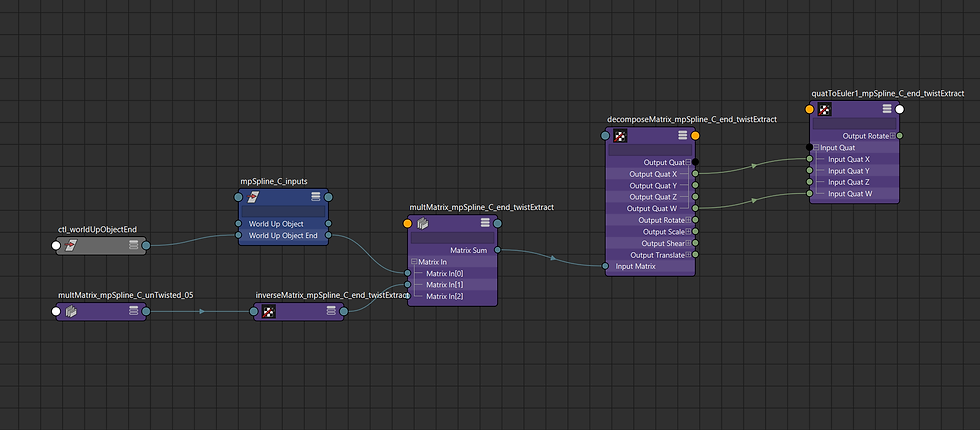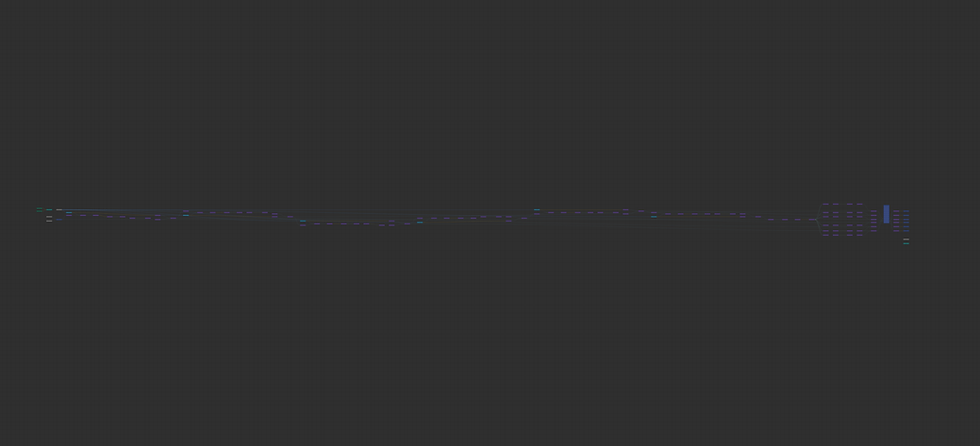top of page# Solving Spline Overshoot

### What's The Problem?

When working with ikSplines in Maya I always run into the same two issues:

1. The first joint in the chain lies secant to the curve instead of tangent, so the mesh does not connect smoothly at the base of the ikSpline.

2. The tip joint does not sit exactly on the edge of the curve, especially when the spline curve bends sharply.

Both of these issues can be resolved by using motionPaths instead of an ikSpline but then we lose the best feature of the ikSpline, the advance twist settings (which is the most robust and stable method of distributing twist along a curve).

But we can actually have the best of both worlds and the nice positioning and orientation from the motionPath and still have the same twist results. To do this we first need to understand how the ikSpline advance twist distribution works.

### How Does Advanced Twist Actually Work?

In this example I am going to assume you are using "Object Rotation Up" but this is true for all "world up types". I'm also not going to go into any math or node networks just yet.

Advanced twist works by first solving for all the positions along the curve.

Then for the first joint in the chain it finds the orientation that aims to the next position without twisting along the primary axis (relative to the the worldUpObject).

Then for the second joint in the chain it finds the orientation that aims to the next position without twisting along the primary axis (relative to the prior joint). This is done for the rest of the joint too.

So what we end up with is a join chain where the chain bends along the curve without twisting. But the orientation of our last joint doesn't match our worldUpObjectEnd.

So the ikSplineSolver finds the relative twist between the last joint and the worldUpObjectEnd, and it distributes that twist along the whole joint chain.

### Can we do that with nodes?

That explanation had a lot of hand waving but now we're going to get into the specifics. I'll do my best to provide resources so that if you aren't familiar with the techniques we are using you can look into them for yourself.

So first let's find the positions. We can use a motionPath, or pointOnSurfaceInfo node. I'm going to use a motion path because no only will it let us find the positions based on a "percent length" instead of parameter values, but also cut down on the number of nodes we need for later steps.

Next we are going to take the orientation from the first motionPath and modify it so that it does not twist relative to our worldUpObject. To do this we are going to be using this twist extraction technique

https://bindpose.com/maya-matrix-nodes-part-2-node-based-matrix-twist-calculator/ .

We are going to extract the twist, reverse it and apply it relative to the orientation from the motionPath.

Here's the overall node network:Then we can perform the same operations for the next motionPath (using the resulting matrix from the first motionPath in place of the worldUpObject):and we'll end up with the same result as the ikSpline, bending along the curve without twisting.

Now we need to figure out what degree of twist we want need to distribute along the curve. I am going to use the the same twist extractor that we just used. In my experience it is more robust than using either an aimConstraint or trying to use the caching functionality of an orientConstraint, but you can easily use another method or just directly plug in a twist value. This twist extractor is limited to 180 degrees but the distribution will work with any value.

So we're going to find the twist of the worldUpObjectEnd relative to matrix from the last object on the chain.

Here's that part of the node network:Then we just need to distribute that extracted twist along the curve by multiplying it by the fraction of the length for each object and then applying it as an offset matrix. Here's that of the node network:Here's the full rig in action:

If you want to mess around with this I have uploaded a script that you can use to build the rig here: